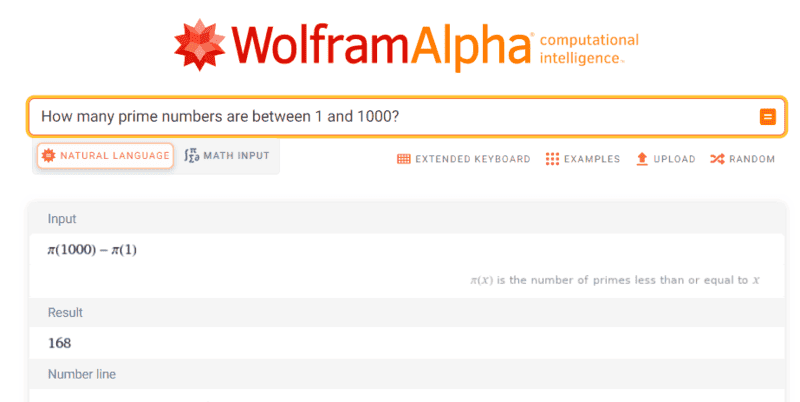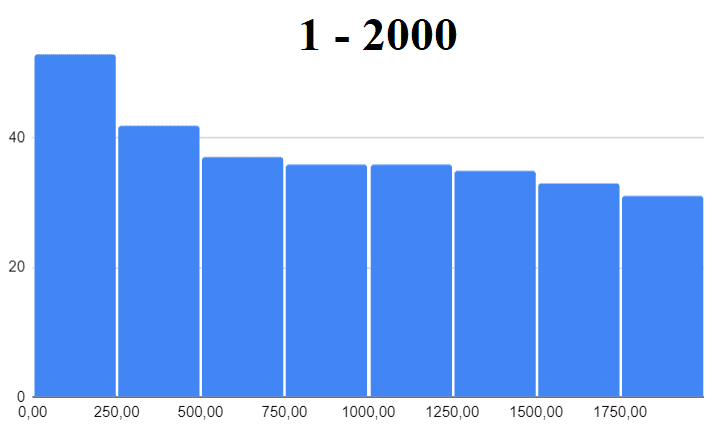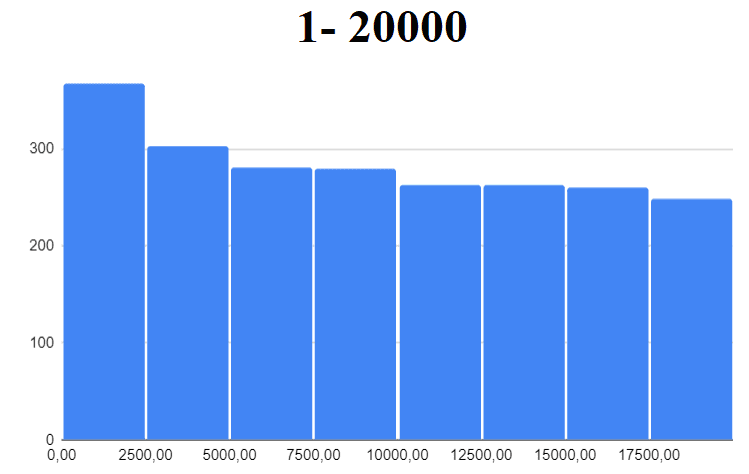# Primes -- Probability that the sum of two random integers is Prime

• I
• donglepuss

#### donglepuss

TL;DR Summary
if i select two integers at random between 1 and 1,000, what is the probability that their sum will be prime?
im thinking i should just integrate (binominal distribution 1-2000 * prime probability function) and divide by integral of bin. distr. 1-2000.

note that I am looking for a novel proof, not just some brute force calculation.

(this isn't homework, I am just curious.)

Any number n is expressed n-1 ways as
$$n=1+(n-1)=2+(n-2)=..=(n-1)+1$$
for n##\leq##N say N=1000. For larger n
$$2000=1000+1000$$
$$1999=1000+999=999+1000$$
...
There are 2N-(n-1) ways of expression

So the probability of you look for could be
$$\frac{\sum_{n=1}^{2N} min\{(n-1), 2N-(n-1)\}p(n)}{\sum_{n=1}^{2N} min\{(n-1), 2N-(n-1)\}}$$$$=\frac{\sum_{n=1}^{N} (n-1)p(n)+\sum_{n=N+1}^{2N} \{2N-(n-1)\}p(n)}{N^2}$$
where p(n)=1 for prime number n and p(n)=0 for otherwise, though I am not sure whether it satisfies randomness you expect or not.

Last edited:
•malawi_glenn
There are 303primes between 1 and 1000, one is even 302 are odd.
2 = 1+1: one possibility
3 = 1+2, 2+1: two possibilities
5= 1+4, 2+3, 3+2, 4+1: four possibilities
7= 1+6, 2+5, 3+4, 4+3, 5+2, 6+1: six positibilities
11 = 1+10, 2+9, 3+8, 4+7, 5+6, 6+5, 7+4, 8+3, 9+2, 10 +1: ten positibilities
Note that the number of possibilities to add two numbers to a prime is ##p## is ##p-1##

In order to perform a formal proof which is not a "brute force" calculation, you need a function that gives the primes between 1 and 2000 (as mentione in the post above). Otherwise you have to do a brute force calculation.

•PeroK
Otherwise you have to do a brute force calculation.
Which is also known as counting. And that's sometimes a useful idea.

Which is also known as counting. And that's sometimes a useful idea.
Yeah, I was just connecting with the opening post nomenclature ;)

For primes ##p \le 1001## there are ##p - 1## ways to get them.

For primes ##1001 < p < 2000## there are ##2001 - p## ways to get them.

•malawi_glenn
probability is ##\dfrac{146178}{1000^2} \approx 14.6\% ##

•anuttarasammyak
probability is ##\dfrac{146178}{1000^2} \approx 14.6\% ##

There are 168 prime numbers between 1 to 1000, 16.8% and 303 prime numbers between 1 to 2000, 15.15%.Last edited:
There are 168 prime numbers between 1 to 1000, 16.8%. So I assume the distribution becomes sparse for larger number region.
But the question was if you draw two numbers 1-1000, is their sum a prime?
You can therefore get primes also between 1001 and 2000...

You also have to take into account of many ways there are to add up numbers to a prime.

The question was not "draw a random number, what is the probability that it is prime?"

Yes the distribution of primes become more and more sparse (##\pi(x)## goes as ##x / \ln(x)## for large ##x##)

As I wrote, there are 303 primes between 1 and 2000
that is 15.15% to be a prime - but again, this is not the answer to the question rasied by the OP.
Between 1 and 3000 is 430 primes, prob that one number is prime is 14.33%

•PeroK and anuttarasammyak
What I said in the previous post has no direct relation to the problem. I apologize confusion it may cause.

What I said in the previous post has no direct relation to the problem. I apologize confusion it may cause.
Then why quoting my post? Two unrelated problems.

I was curious to know the difference on the results of "sum of two numbers are prime" and "a number is prime". Again I apologize my digression.

•malawi_glenn
More results

Drawing two numbers 1-100, their sum is prime: 22.9%
Drawing two numbers 1-500, their sum is prime: 16.2%
Drawing two numbers 1-1000, their sum is prime: 14.6%
Drawing two numbers 1-5000, their sum is prime: 11.9%
Drawing two numbers 1-10000, their sum is prime: 11.0%

More results

Drawing two numbers 1-100, their sum is prime: 22.9%
Drawing two numbers 1-500, their sum is prime: 16.2%
Drawing two numbers 1-1000, their sum is prime: 14.6%
Drawing two numbers 1-5000, their sum is prime: 11.9%
Drawing two numbers 1-10000, their sum is prime: 11.0%
Compare with the prime counting function ##\pi(n)##:

 n pi(n) 100​ 21.71%​ 500​ 16.09%​ 1000​ 14.48%​ 5000​ 11.74%​ 10000​ 10.86%​

•malawi_glenn
Yeah, it is very close.

Should be a reason for that.

Judging from the prime number distrubutions:The left- and rightmost bins contribute very little to the overall sum of favorable outcomes.
For 1-20000 those two bins contribute 15.6%, and that is 0.156×0.11 = 0.017 = 1.7%

There is an elegant closed form solution if you limit the problem to only even prime numbers …

•jbriggs444
Yeah, it is very close.

Should be a reason for that.
Although the sum of two integers has a bell-shaped distribution, ultimately it does not prefer primes to non-primes or vice versa. You would, therefore, expect it to be close to the probability that a single integer at the mid-point of the distribution is prime.

•malawi_glenn
Compare with the prime counting function ##\pi(n)##:

 n pi(n) 100​ 21.71%​
Wait a minute. There are 25 primes less than 100. Yet you quote 21.71 percent. What's the deal? Surely 25% of the integers in the range [1 - 100] are prime.

•PeroK
Wait a minute. There are 25 primes less than 100. Yet you quote 21.71 percent. What's the deal? Surely 25% of the integers in the range [1 - 100] are prime.
I just used the asymptotic limit ##\frac 1 {\ln(n)}##.

I just used the asymptotic limit ##\frac 1 {\ln(n)}##.
So this was not, in fact, quoting correct values for ##\pi(n)##. This, in turn, casts doubt on the significance of a comparison between the quoted values and the probability of primeness for a particular triangular distribution of small finite numbers.

Integrating the product of a high-at-the-ends function (the incremental prime counting function) and a high-in-the-middle function (the distribution of the sum of two d100's) should yield a result that is lower than the product of two more nearly uniform distributions with the same mean values.

•malawi_glenn
So this was not, in fact, quoting correct values for ##\pi(n)##.
I forgot I picked the approximation, because I was thinking about large ##n##. I would expect the sum of two integers to converge to the prime counting function for large ##n##. Interesting, it seems to stick quite close to ##\frac 1 {\ln(n)}##. We need data for a larger ##n## in any case:

 n 1/ln(n) pi(n)/n 100​ 22.9%​ 21.7%​ 25.0%​ 500​ 16.2%​ 16.1%​ 19.0%​ 1000​ 14.6%​ 14.5%​ 16.8%​ 5000​ 11.9%​ 11.7%​ 13.4%​ 10000​ 11.0%​ 10.9%​ 12.3%​

•malawi_glenn
This is as high as I can go with my list of the first 100 million primes. It seems to converge more to the logarithmic approximation than to the counting function itself.

 n Answer 1/ln(n) pi(n)/n 100,000​ 8.800%​ 8.686%​ 9.592%​ 1,000,000​ 7.306%​ 7.238%​ 7.850%​ 5,000,000​ 6.538%​ 6.483%​ 6.970%​ 10,000,000​ 6.255%​ 6.204%​ 6.646%​ 49,999,990​ 5.682%​ 5.641%​ 6.002%​

Last edited:
•jbriggs444 and malawi_glenn
I forgot I picked the approximation, because I was thinking about large ##n##.
You need to remember just how bad that approximation is for any practical value of ## n ## (the error is greater than 1.5% for all n less than ## 10^{29} ##).

note that I am looking for a novel proof, not just some brute force calculation.

(this isn't homework, I am just curious.)
As you can infer from the mistaken assumptions followed through above, the irregularity of the prime counting function means that there can never be a "novel proof", the only way to calculate the probability exactly for any ## n ## is brute force (i.e. by summing over a number of terms not less than ## \pi(n) ##).

This is as high as I can go with my list of the first 100 million primes. It seems to converge more to the logarithmic approximation than to the counting function itself.
I had alluded to an expected skew based on the shape of the distributions. Let us try to see how that effect plays out. Rather than ##\pi(n)##, we will use the more nicely behaved ##\frac{1}{\ln(n)}## prime density function.

What is the probability that 2d100 will yield a "prime" result based on this "prime" density distribution? That should be$$\frac{1}{10000} (\sum_{n=2}^{101} \frac{n-1}{\ln(n)}+ \sum_{n=102}^{200} \frac{201-n}{\ln(n)})$$
What is the probability that 1d199+1 will yield a "prime" result based on this prime density distribution? That should be$$\frac{1}{199} (\sum_{n=2}^{200} \frac{1}{\ln(n)})$$
Compare this to the result expected from 2d100 (or d199+1) with a uniform "prime" density inferred from the density at the midpoint of the distribution. That should be$$\frac{1}{\ln(101)}$$
So yes there is a skew. As expected, the product of two distributions, one concave upward and one concave downward is less than the product of a concave upward distribution and a uniform distribution. Also, the mean of a concave up distribution is greater than its midpoint value.
my code said:
C:\Users\John\Documents>primes.pl
2d100: 0.22610721620634
1d199+1: 0.251473806850781
Uniform prime density: 0.216679065335532

C:\Users\John\Documents>primes.pl
2d1000: 0.148260310578937
1d1999+1: 0.15739459190828
Uniform prime density: 0.144743883947654

C:\Users\John\Documents>primes.pl
2d10000: 0.110363723394825
1d19999+1: 0.114426785937757
Uniform prime density: 0.108572441724441

C:\Users\John\Documents>primes.pl
2d100000: 0.0879394496681733
1d199999+1: 0.0901797001323427
Uniform prime density: 0.0868588209364143

C:\Users\John\Documents>primes.pl
2d1000000: 0.0731041904157329
1d1999999+1: 0.0745273494626591
Uniform prime density: 0.0723824084113312

C:\Users\John\Documents>primes.pl
2d10000000: 0.0625579197454417
1d19999999+1: 0.0635452443175609
Uniform prime density: 0.0620420684583999

C:\Users\John\Documents>primes.pl
2d100000000: 0.0546736999176157
1d199999999+1: 0.0553998734542306
Uniform prime density: 0.0542868102084359

C:\Users\John\Documents>primes.pl
2d1000000000: 0.0485557820044112
1d1999999999+1: 0.0491126509835289
Uniform prime density: 0.0482549424313661
Edit: made the number of sides on the dice into a parameter and re-ran a few times. The code really starts to strain at 100 million. Took a couple minutes. A billion took more like twenty minutes.

With 64 bit floats, we are probably starting to tickle the edges of precision loss at 1 billion. Adding up a column of a billion or two small numbers, some almost a factor of a billion larger than others calls for 18 digits and we only have 16 or 17. If I wanted to do things right, I'd have been summing the columns in order from low to high to minimize that particular problem. Doing sub-totals would work even better. It might have also been worth merging the separate loops, buying a factor of two on the redundant calls to log(). [But Kernighan and Plauger lesson 1: "Write clearly; don't be too clever"]

That's before doing any of that mathematician magic stuff, looking for a better approximate formula or a way to telescope the series.

Bat Signal to @PeroK -- take a look at the extra results editted in.

Last edited:
•PeroK
What happens as ##N \to \infty##?

•jbriggs444
What happens as ##N \to \infty##?
Sadly, I am more of a programmer than a mathematician.

Sadly, I am more of a programmer than a mathematician.
False modesty!

•jbriggs444